• +91 9971497814
• info@interviewmaterial.com

# RD Chapter 12- Heron-s Formula Ex-12.5 Interview Questions Answers

### Related Subjects

Question 1 : ABC is a triangle and D is the mid-point of BC. The perpendiculars from D to AB and AC are equal. Prove that the triangle is isosceles.

Given : In ∆ABC, D is mid-point of BC and DE ⊥ AB, DF ⊥ AC and DE = DF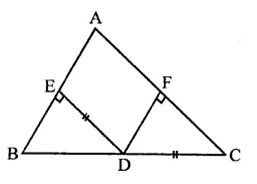To Prove : ∆ABC is an isosceles triangle
Proof : In right ∆BDE and ∆CDF,
Side DE = DF
Hyp. BD = CD
∴ ∆BDE ≅ ∆CDF (RHS axiom)
∴ ∠B = ∠C (c.p.c.t.)
Now in ∆ABC,
∠B = ∠C (Prove)
∴ AC = AB (Sides opposite to equal angles)
∴ AABC is an isosceles triangle

Question 2 : ABC is a triangle in which BE and CF are, respectively, the perpendiculars to the sides AC and AB. If BE = CF, prove that ∆ABC is an isosceles.

Given : In ∆ABC,
BE ⊥ AC and CF ⊥ AB
BE = CF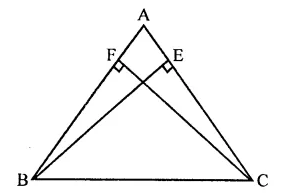To prove : AABC is an isosceles triangle
Proof : In right ABCE and ABCF Side
BE = CF (Given)
Hyp. BC = BC (Common)
∴ ∆BCE ≅ ∆BCF (RHS axiom)
∴ ∠BCE = ∠CBF (c.p.c.t.)
∴ AB = AC (Sides opposite to equal angles)
∴ ∆ABC is an isosceles triangle

Question 3 : If perpendiculars from any point within an angle on its arms are congruent, prove that it lies on the bisector of that angle.

Given : A point P lies in the angle ABC and PL ⊥ BA and PM ⊥ BC and PL = PM. PB is joined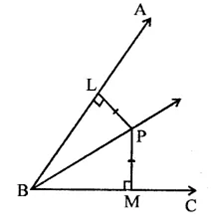To prove : PB is the bisector ∠ABC,
Proof : In right ∆PLB and ∆PMB
Side PL = PM (Given)
Hyp. PB = PB (Common)
∴ ∆PLB ≅ ∆PMB (RHS axiom)
∴ ∆PBL = ∆PBM (c.p.c.t.)
∴ PB is the bisector of ∠ABC

Question 4 : In the figure, AD ⊥ CD and CB ⊥ CD. If AQ = BP and DP = CQ, prove that ∠DAQ = ∠CBP.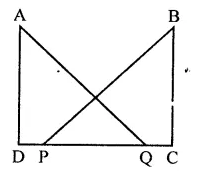Given : In the figure,
AD ⊥ CD and CB ⊥ CD, AQ = BP and DP = CQ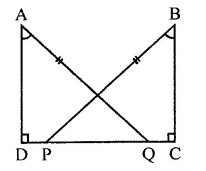To prove : ∠DAQ = ∠CBP
Proof : ∵ DP = CQ
∴ DP + PQ = PQ + QC
⇒ DQ = PC
Now in right ∆ADQ and ∆BCP
Side DQ = PC (Proved)
Hyp. AQ = BP
∴ ∆ADQ ≅ ∆BCP (RHS axiom)
∴ ∠DAQ = ∠CBP (c.p.c.t.)

Question 5 :
Which of the following statements are true (T) and which are false (F):
(i) Sides opposite to equal angles of a triangle may be unequal.
(ii) Angles opposite to equal sides of a triangle are equal.
(iii) The measure of each angle of an equilateral triangle is 60°.
(iv) If the altitude from one vertex of a triangle bisects the opposite side, then the triangle may be isosceles.
(v) The bisectors of two equal angles of a triangle are equal.
(vi) If the bisector of the vertical angle of a triangle bisects the base, then the triangle may be isosceles.
(vii) The two altitudes corresponding to two equal sides of a triangle need not be equal.
(viii)If any two sides of a right triangle are respectively equal to two sides of other right triangle, then the two triangles are congruent.
(ix) Two right triangles are congruent if hypotenuse and a side of one triangle are respectively equal to the hypotenuse and a side of the other triangle.

(i) False : Sides opposite to equal angles of a triangle are equal.
(ii) True.
(iii) True.
(iv) False : The triangle is an isosceles triangle.
(v) True.
(vi) False : The triangle is an isosceles.
(vii) False : The altitude an equal.
(viii) False : If one side and hypotenuse of one right triangle on one side and hypotenuse of the other right triangle are equal, then triangles are congruent.
(ix) True.

Question 6 :
Fill in the blanks in the following so that each of the following statements is true.
(i) Sides opposite to equal angles of a triangle are …….
(ii) Angle opposite to equal sides of a triangle are …….
(iii) In an equilateral triangle all angles are …….
(iv) In a ∆ABC if ∠A = ∠C, then AB = …….
(v) If altitudes CE and BF of a triangle ABC are equal, then AB = ……..
(vi) In an isosceles triangle ABC with AB = AC, if BD and CE are its altitudes, then BD is ……… CE.
(vii) In right triangles ABC and DEF, if hypotenuse AB = EF and side AC = DE, then ∆ABC ≅ ∆……

(i) Sides opposite to equal angles of a triangle are equal.
(ii) Angle opposite to equal sides of a triangle are equal.
(iii) In an equilateral triangle all angles are equal.
(iv) In a ∆ABC, if ∠A = ∠C, then AB = BC.
(v) If altitudes CE and BF of a triangle ABC are equal, then AB = AC.
(vi) In an isosceles triangle ABC with AB = AC, if BD and CE are its altitudes, then BD is equal to CE.
(vii) In right triangles ABC and DEF, it hypotenuse AB = EF and side AC = DE, then ∆ABC ≅ ∆EFD.

Question 7 : ABCD is a square, X and Y are points on sides AD and BC respectively such that AY = BX. Prove that BY = AX and ∠BAY = ∠ABX.

Given : In square ABCD, X and Y are points on side AD and BC respectively and AY = BX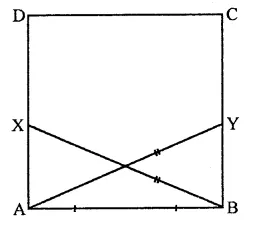To prove : BY = AX
∠BAY = ∠ABX
Proof: In right ∆BAX and ∆ABY
AB =AB (Common)
Hyp. BX = AY (Given)
∴ ∆BAX ≅ ∆ABY (RHS axiom)
∴ AX = BY (c.p.c.t.)
∠ABX = ∠BAY (c.p.c.t.)
Hence, BY = AX and ∠BAY = ∠ABX.

Todays Deals### RD Chapter 12- Heron-s Formula Ex-12.5 Contributorskrishan

Name:
Email:

# Latest News# 9000 interview questions in different categories